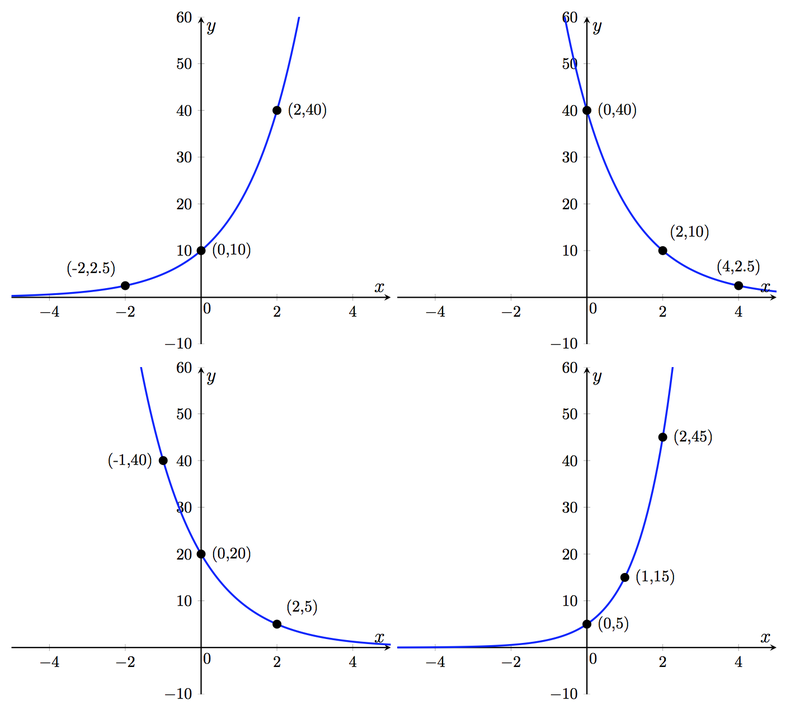# Identifying Exponential Functions

Alignments to Content Standards: F-IF.C.7.e F-LE.A.2

An exponential function is a function of the form $f(x)=ab^x$ for positive real numbers $a$ and $b$.

1. Use the app below to sketch exponential functions for various values of $a$ and $b$. Describe in words the effect of changing $a$ and $b$ on the shape of the graph.

2. Find a function of the form $f(x)=a\cdot b^x$ for each of the four graphs below.## IM Commentary

The task is an introduction to the graphing of exponential functions. The first part asks students to use technology to experiment with the two parameters defining an exponential function, with little guidance.  Since it is important for the second part, teachers should encourage students to try a wide range of values, and in particular, values of $b$ both less than and greater than 1. The task includes a desmos appe, in which students can make use of sliders to more viscerally see the effect of changing $a$ and $b$ separately. It may not be clear at first glance, for example, that, while increasing, $b$ can greatly affect the "steepness" of the graph it does not affect its $y$-intercept.

The stem of the task does not directly use the terms "exponential growth" or "exponential decay", though this may serve as a convenient place to introduce them in a lesson, as students begin to independently observe the stark differences between the cases $b>1$ and $0<b<1$.

The solution does not prescribe one method for determining algebraic expressions for the graphs. Instructors may wish to continue letting students use graphing software, or have them start practicing the algebraic skill of identifying an exponential function by choosing points. For example, in the top-left graph, we identify that the $y$-intercept is $a=10$, and then the input-output pair $(2,40)$ implies that $10\cdot b^2=40$, which is easily seen to require $b=2$.

## Solution

1. There are many things to observe here!  For one, it appears that sliding the value of $b$ affects the rate at which the graph increases for $x>0$ (and decreases for $x<0$), but leaves the $y$-intercept unchanged.  This can be seen algebraically by noting that since $f(x)=ab^x$, we always have $f(0)=a$.  So the $y$-intercept is governed by $a$ alone, and we see that $a$ directly represents the $y$-intercept of the graph. Sliding the value of $a$ also reveals the transformative effect of that parameter -- it's easiest to interpret $a$ as a vertical stretch of the graph by a factor of $a$.  On the other hand, $b$ affects the rate at which the function increases or decreases. A high value of $b$ has a graph that gets very large very quickly. On the other hand, values when $0<b<1$ have a drastically different appearance, with the graph decreasing as $x$ increases. The striking difference between the cases $b>1$ and $0<b<1$ is given a name when $f$ models a real-world quantity -- when $b>1$ we say that the function exhibits exponential growth, and when $0<b<1$ the function exhibits exponential decay.  It's also slightly interesting to note that when $b=1$, the exponential function is simply the constant function $f(x)=a$, and so serves as a sort of neutral zone between exponential growth and exponential decay.
2. The four functions, from top left to bottom right, are:
• $f(x)=10\cdot 2^x$
• $f(x)=40\cdot \left(\frac{1}{2}\right)^x$
• $f(x)=20\cdot \left(\frac{1}{2}\right)^x$
• $f(x)=5\cdot 3^x$